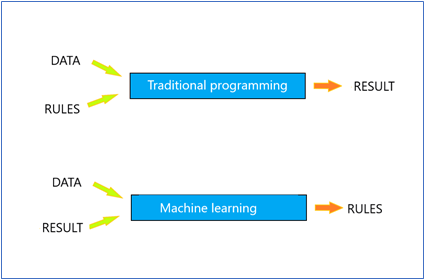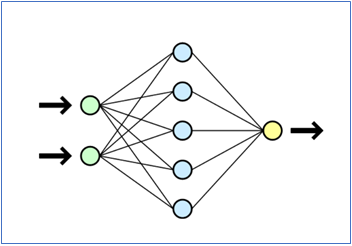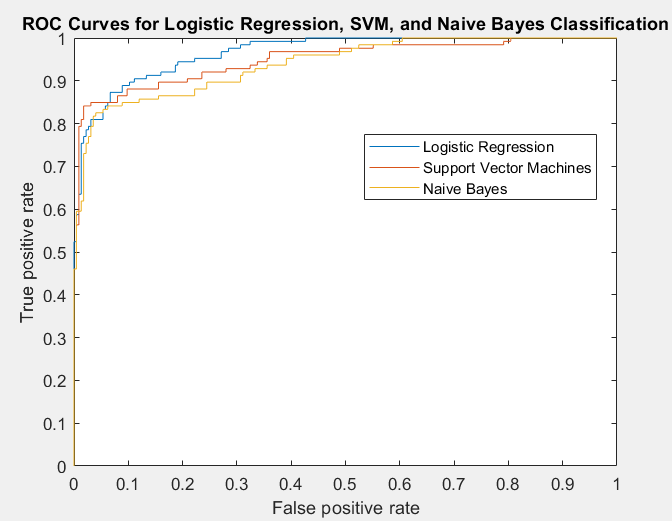# Basics of machine learning

Machine learning is a genre of Artificial Intelligence (AI), which uses algorithms that provide systems the ability to automatically learn from a dataset (such as X-rays or health data containing tables) and improve from experience, without being explicitly programmed.  The aim is to look for patterns in data and often also make it possible to train a computer model for identifying or predicting certain conditions.

Machine learning algorithms can be divided into two categories. In supervised learning the data items in the dataset are labeled with the correct answer:  e.g. some data items (images, rows in a table etc.) labeled as having the disease and the other items labeled as not having it. A suitable machine learning algorithm uses the data to build general models to map the data to the correct answer. This kind of approach can be used to generate e.g. model for recognizing diabetic retinopathy from retinal fundus images or identifying osteoarthritis in CET scans.

In unsupervised learning the data items are not labeled, and the algorithm learns from the data without having correct answers. Unsupervised learning can be used for clustering: finding clusters of similar data items. Often this type of analysis is done in data mining projects, where hidden patterns of data are looked for.

### Basic architecture of neural networks

Performing machine learning involves creating a model, which is trained on the training data, thereby achieving capability to process new data to make classification and prediction tasks. One of the most important type of models is artificial neural network, which was inspired by biological neural networks of the brain.

In practice artificial neural networks are implemented as computer programs. The difference between machine learning and traditional rule-base programming is illustrated in the figure below describing classification task. In supervised machine learning the program uses data to make the rules for classification in the training phase, while in traditional programming the rules are explicitly programmed by humans to get the classification task done.  In machine learning the program learns from the labeled data.Fig.1 Basic difference between classification task performed by traditional programs (above) and by machine learning (training phase) below. After the training phase, the rules can be applied to new data for classification.

Artificial neural networks exist in many types of varieties, but in one of the simplest forms, the architecture consists of only three layers:

• input layer

• hidden layer (one or many layers)

• output layer

Each layer consists of neurons, which function as nodes receiving inputs and sending them to the next layer according to the rules of the algorithm. Each neuron has a weight (in Finnish painokerroin) by which the input can be strengthened or diminished before sending to the next layer.

Neural network is established by connecting the neurons together, as shown in Figure 2. The weights of the neurons can be adjusted during the training phase of the neural network.The number of hidden layers varies widely. In deep learning models, the input data is passed through a series of nonlinear transformations before it becomes output.  The term “deep” refers to the number of hidden layers in the neural network. While traditional neural networks contain only 2-3 hidden layers, deep networks can have as many as 100-150 of them. Deep learning can achieve better recognition accuracy than other machine learning models, but it requires extremely large amounts of labeled data and substantial computing power.

### Dividing dataset to training and testing data

In supervised machine learning, the available data is divided into two datasets: training data and testing data.  Training data is used to train the model and the test set is used to evaluate the performance of the model by calculating error metrics. In order to get an unbiased estimate of performance, it is important that the test set is kept separate from the training data. If the test set would contain examples from the training set, it would be difficult to assess whether the model has learned to generalize from the training data or has just learned to memorize it.

Sometimes even a third dataset called validation data (or hold-out dataset) is needed.  It is used to tune variables called hyperparameters, which control how the model is learning. With validation data it is possible to fine-tune a model’s parameters and select the best performing model. However, not all machine learning algorithms need a validation set.

How is the data divided into training and test datasets?

Dataset sizes vary widely and so do the practices of data division into training and testing datasets. When there are lots of data (thousands-millions-billions observations), it is common to allocate i.e. 50 % of the data to the training set, 25 percent to the test set, and the remainder to the validation set. With moderate dataset size, division of 70-80% for training data and 20-30% for testing data is also used.

When data is scarce, a resampling method called cross-validation can be used.  The data is partitioned, e.g. 80% for training and 20% for testing.  After that, the same original data is divided again into new training and testing sets, the model is iteratively trained and validated on these differently allocated sets. This is done in iterations several times to get a reliable estimate of the model’s performance.

### How accurate is the prediction model?

There are a variety of performance metrics you can choose from.  The choice depends on a specific machine learning task to be performed, such as classification, regression, prediction, clustering etc.  Some metrics are specific to a task while others, such as precision-recall, are useful for multiple tasks.

Here are some widely used performance metrics for supervised learning tasks:

Classification Accuracy is the number of correct predictions as a ratio of all predictions, such as 60% or 97%.  It works well as a performance metrics only if there are equal number of samples belonging to each class. This is often not the case in medical prediction problems, where the frequency of a diseased state is rarer than the healthy state. Furthermore, the cost of misdiagnosing a sick person as healthy is higher than sending a healthy person to more tests. Classification accuracy should therefore be used together with other performance metrics, such as the number of false positives and false negatives presented in a confusion matrix. From the matrix, several performance metrics can be calculated, e.g.

Sensitivity is the number of items correctly identified as positive out of total true positives.

Specificity is the number of items correctly identified as negative out of total negatives.

See more about confusion matrix and other performance metrics in

Area Under Curve (AUC) is a great visualization tool for presenting performance metrics. It is also known as ROC (Receiver Operating Curve). The value range is 0.5 -1.0: the higher the AUC, the better the model is at classification, e.g. at distinguishing between patients with disease or no disease. In ROC curve the true positive rate is shown on the y axis while false positive rate is shown on the x axis, as exemplified in the figure below.Fig.3 Example of ROC curves: same data analyzed with different algorithms. In this example, AUC is 0.97, 0.95 or 0.94, depending on the used algorithm. The dataset was iris dataset from Matlab, and the code for ROC is published in Mathworks as an example code: https://se.mathworks.com/matlabcentral/fileexchange/65629-example-matlab-script-to-plot-roc-curve-for-different-classification-algorithms).

Last updated: 12.3.2020